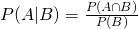# Conditional ProbabilitySome students complain that they often read a question and cannot figure if there is a conditional probability present. So lets hope the following explanation will aid them to better understand it. Lets state the formula first.Probability is always Counter Intuitive.

How does that work? I will first tell students that B is usually the event that had occurred, it is a prior information to A occurring. Other words,is really the probability that A will happen, knowing B had occurred. Consider A to be the event you go to the beach and B is the event the newscaster announce thunderstorm. After B occurred, the chances (probability) of you doing A, will definitely decrease.

So keywords to look out for in A-levels, “if, given, knowing”.

Not readable? Change text.